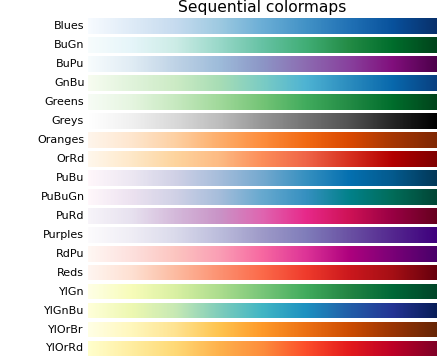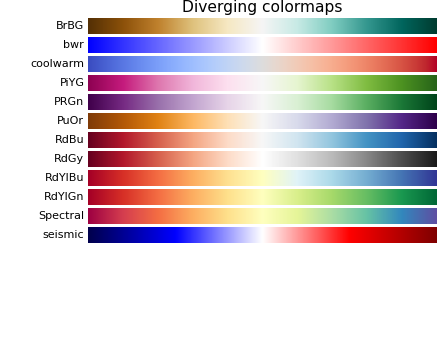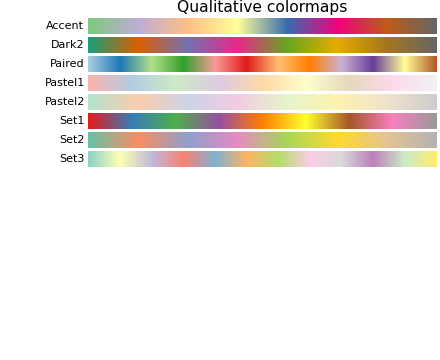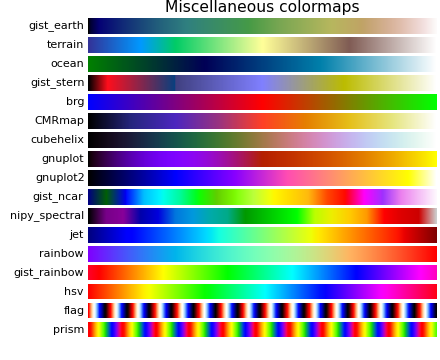### We're updating the default styles for Matplotlib 2.0

Learn what to expect in the new updates#### Previous topic

color example code: color_cycle_demo.py

#### Next topic

color example code: named_colors.py

# color example code: colormaps_reference.py¶(png, hires.png, pdf)(png, hires.png, pdf)(png, hires.png, pdf)(png, hires.png, pdf)(png, hires.png, pdf)

```"""
Reference for colormaps included with Matplotlib.

This reference example shows all colormaps included with Matplotlib. Note that
any colormap listed here can be reversed by appending "_r" (e.g., "pink_r").
These colormaps are divided into the following categories:

Sequential:
These colormaps are approximately monochromatic colormaps varying smoothly
between two color tones---usually from low saturation (e.g. white) to high
saturation (e.g. a bright blue). Sequential colormaps are ideal for
representing most scientific data since they show a clear progression from
low-to-high values.

Diverging:
These colormaps have a median value (usually light in color) and vary
smoothly to two different color tones at high and low values. Diverging
colormaps are ideal when your data has a median value that is significant
(e.g.  0, such that positive and negative values are represented by
different colors of the colormap).

Qualitative:
These colormaps vary rapidly in color. Qualitative colormaps are useful for
choosing a set of discrete colors. For example::

color_list = plt.cm.Set3(np.linspace(0, 1, 12))

gives a list of RGB colors that are good for plotting a series of lines on
a dark background.

Miscellaneous:
Colormaps that don't fit into the categories above.

"""
import numpy as np
import matplotlib.pyplot as plt

cmaps = [('Sequential',     ['Blues', 'BuGn', 'BuPu',
'GnBu', 'Greens', 'Greys', 'Oranges', 'OrRd',
'PuBu', 'PuBuGn', 'PuRd', 'Purples', 'RdPu',
'Reds', 'YlGn', 'YlGnBu', 'YlOrBr', 'YlOrRd']),
('Sequential (2)', ['afmhot', 'autumn', 'bone', 'cool', 'copper',
'gist_heat', 'gray', 'hot', 'pink',
'spring', 'summer', 'winter']),
('Diverging',      ['BrBG', 'bwr', 'coolwarm', 'PiYG', 'PRGn', 'PuOr',
'RdBu', 'RdGy', 'RdYlBu', 'RdYlGn', 'Spectral',
'seismic']),
('Qualitative',    ['Accent', 'Dark2', 'Paired', 'Pastel1',
'Pastel2', 'Set1', 'Set2', 'Set3']),
('Miscellaneous',  ['gist_earth', 'terrain', 'ocean', 'gist_stern',
'brg', 'CMRmap', 'cubehelix',
'gnuplot', 'gnuplot2', 'gist_ncar',
'nipy_spectral', 'jet', 'rainbow',
'gist_rainbow', 'hsv', 'flag', 'prism'])]

nrows = max(len(cmap_list) for cmap_category, cmap_list in cmaps)
gradient = np.linspace(0, 1, 256)
gradient = np.vstack((gradient, gradient))

def plot_color_gradients(cmap_category, cmap_list):
fig, axes = plt.subplots(nrows=nrows)
fig.subplots_adjust(top=0.95, bottom=0.01, left=0.2, right=0.99)
axes.set_title(cmap_category + ' colormaps', fontsize=14)

for ax, name in zip(axes, cmap_list):
ax.imshow(gradient, aspect='auto', cmap=plt.get_cmap(name))
pos = list(ax.get_position().bounds)
x_text = pos - 0.01
y_text = pos + pos/2.
fig.text(x_text, y_text, name, va='center', ha='right', fontsize=10)

# Turn off *all* ticks & spines, not just the ones with colormaps.
for ax in axes:
ax.set_axis_off()

for cmap_category, cmap_list in cmaps:
plot_color_gradients(cmap_category, cmap_list)

plt.show()
```

Keywords: python, matplotlib, pylab, example, codex (see Search examples)